# Sequences and Summations Section 2 4 Section Summary

• Slides: 29Sequences and Summations Section 2. 4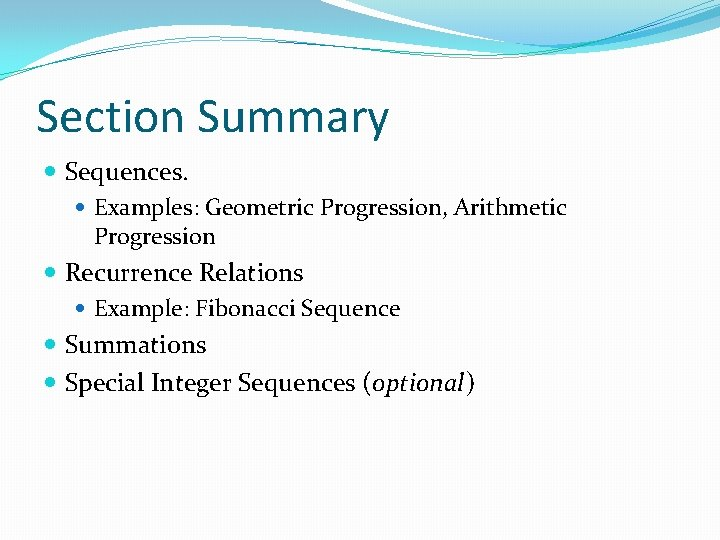Section Summary Sequences. Examples: Geometric Progression, Arithmetic Progression Recurrence Relations Example: Fibonacci Sequence Summations Special Integer Sequences (optional)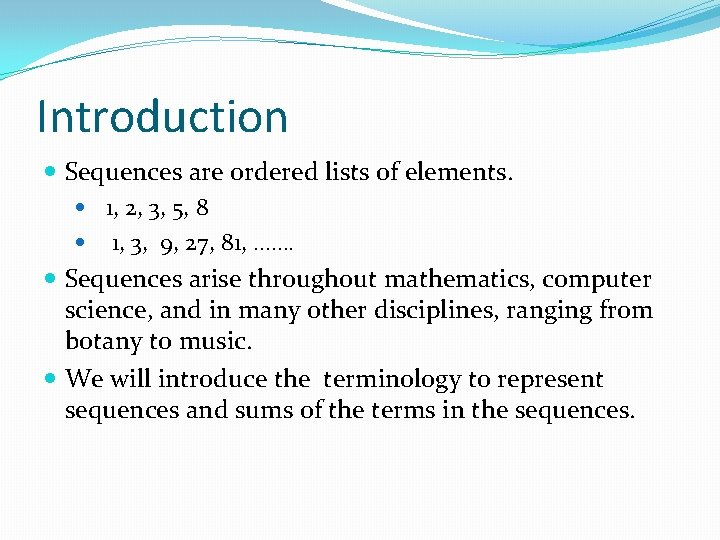Introduction Sequences are ordered lists of elements. 1, 2, 3, 5, 8 1, 3, 9, 27, 81, ……. Sequences arise throughout mathematics, computer science, and in many other disciplines, ranging from botany to music. We will introduce the terminology to represent sequences and sums of the terms in the sequences.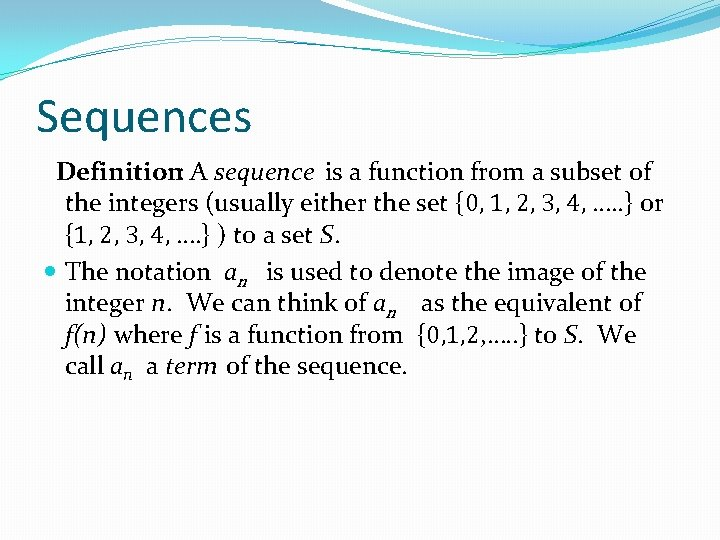Sequences Definition: A sequence is a function from a subset of the integers (usually either the set {0, 1, 2, 3, 4, …. . } or {1, 2, 3, 4, …. } ) to a set S. The notation an is used to denote the image of the integer n. We can think of an as the equivalent of f(n) where f is a function from {0, 1, 2, …. . } to S. We call an a term of the sequence.Sequences Example: Consider the sequence whereGeometric Progression Definition: A geometric progression is a sequence of the form: where the initial term a and the common ratio r are real numbers. Examples: 1. Let a = 1 and r = − 1. Then: 2. Let a = 2 and r = 5. Then: 3. Let a = 6 and r = 1/3. Then: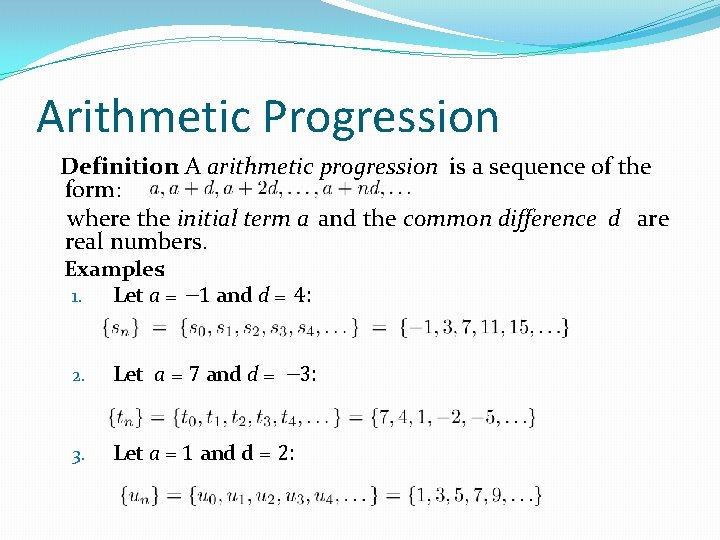Arithmetic Progression Definition: A arithmetic progression is a sequence of the form: where the initial term a and the common difference d are real numbers. Examples: 1. Let a = − 1 and d = 4: 2. Let a = 7 and d = − 3: 3. Let a = 1 and d = 2: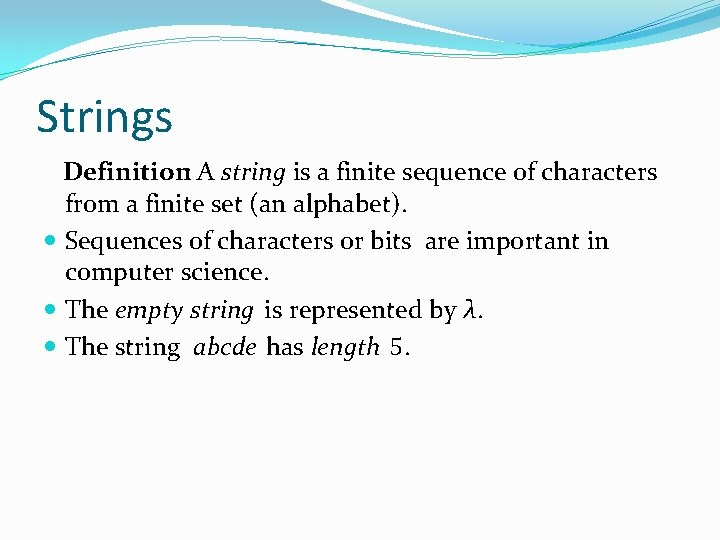Strings Definition: A string is a finite sequence of characters from a finite set (an alphabet). Sequences of characters or bits are important in computer science. The empty string is represented by λ. The string abcde has length 5.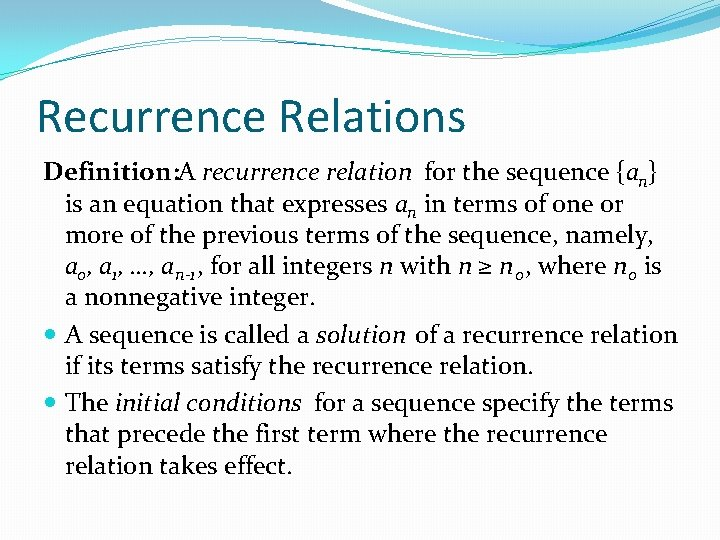Recurrence Relations Definition: A recurrence relation for the sequence {an} is an equation that expresses an in terms of one or more of the previous terms of the sequence, namely, a 0, a 1, …, an-1 , for all integers n with n ≥ n 0, where n 0 is a nonnegative integer. A sequence is called a solution of a recurrence relation if its terms satisfy the recurrence relation. The initial conditions for a sequence specify the terms that precede the first term where the recurrence relation takes effect.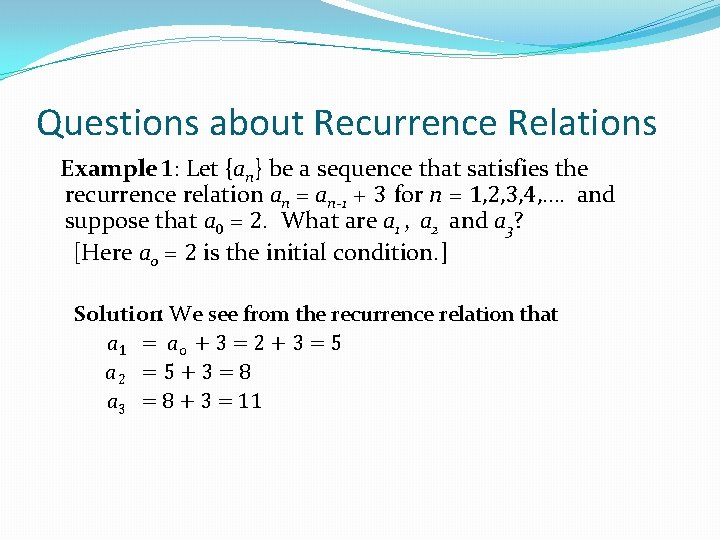Questions about Recurrence Relations Example 1: Let {an} be a sequence that satisfies the recurrence relation an = an-1 + 3 for n = 1, 2, 3, 4, …. and suppose that a 0 = 2. What are a 1 , a 2 and a 3? [Here a 0 = 2 is the initial condition. ] Solution: We see from the recurrence relation that a 1 = a 0 + 3 = 2 + 3 = 5 a 2 = 5 + 3 = 8 a 3 = 8 + 3 = 11Questions about Recurrence Relations Example 2: Let {an} be a sequence that satisfies the recurrence relation an = an-1 – an-2 for n = 2, 3, 4, …. and suppose that a 0 = 3 and a 1 = 5. What are a 2 and a 3? [Here the initial conditions are a 0 = 3 and a 1 = 5. ] Solution: We see from the recurrence relation that a 2 = a 1 - a 0 = 5 – 3 = 2 a 3 = a 2 – a 1 = 2 – 5 = – 3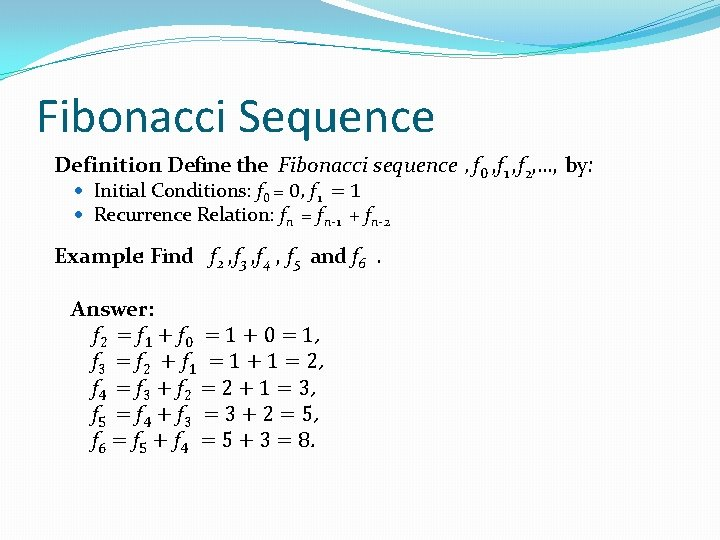Fibonacci Sequence Definition: Define the Fibonacci sequence , f 0 , f 1 , f 2, …, by: Initial Conditions: f 0 = 0, f 1 = 1 Recurrence Relation: fn = f n-1 + f n-2 Example: Find f 2 , f 3 , f 4 , f 5 and f 6. Answer: f 2 = f 1 + f 0 = 1 + 0 = 1, f 3 = f 2 + f 1 = 1 + 1 = 2, f 4 = f 3 + f 2 = 2 + 1 = 3, f 5 = f 4 + f 3 = 3 + 2 = 5, f 6 = f 5 + f 4 = 5 + 3 = 8.Solving Recurrence Relations Finding a formula for the nth term of the sequence generated by a recurrence relation is called solving the recurrence relation. Such a formula is called a closed formula. Various methods for solving recurrence relations will be covered in Chapter 8 where recurrence relations will be studied in greater depth. Here we illustrate by example the method of iteration in which we need to guess the formula. The guess can be proved correct by the method of induction (Chapter 5).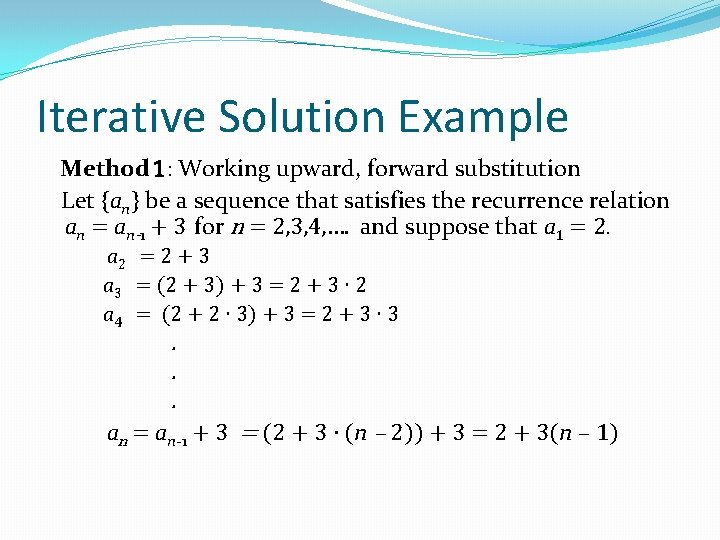Iterative Solution Example Method 1: Working upward, forward substitution Let {an} be a sequence that satisfies the recurrence relation an = an-1 + 3 for n = 2, 3, 4, …. and suppose that a 1 = 2. a 2 = 2 + 3 a 3 = (2 + 3) + 3 = 2 + 3 ∙ 2 a 4 = (2 + 2 ∙ 3) + 3 = 2 + 3 ∙ 3 . . . an = an-1 + 3 = (2 + 3 ∙ (n – 2)) + 3 = 2 + 3(n – 1)Iterative Solution Example Method 2: Working downward, backward substitution Let {an} be a sequence that satisfies the recurrence relation an = an-1 + 3 for n = 2, 3, 4, …. and suppose that a 1 = 2. an = an-1 + 3 = (an-2 + 3) + 3 = an-2 + 3 ∙ 2 = (an-3 + 3 )+ 3 ∙ 2 = an-3 + 3 ∙ 3 . . . = a 2 + 3(n – 2) = (a 1 + 3) + 3(n – 2) = 2 + 3(n – 1)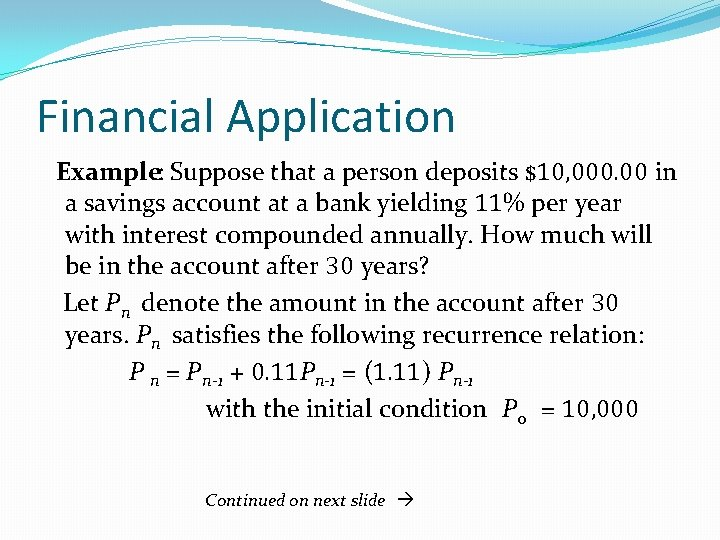Financial Application Example: Suppose that a person deposits \$10, 000. 00 in a savings account at a bank yielding 11% per year with interest compounded annually. How much will be in the account after 30 years? Let Pn denote the amount in the account after 30 years. Pn satisfies the following recurrence relation: P n = P n-1 + 0. 11 Pn-1 = (1. 11) Pn-1 with the initial condition P 0 = 10, 000 Continued on next slide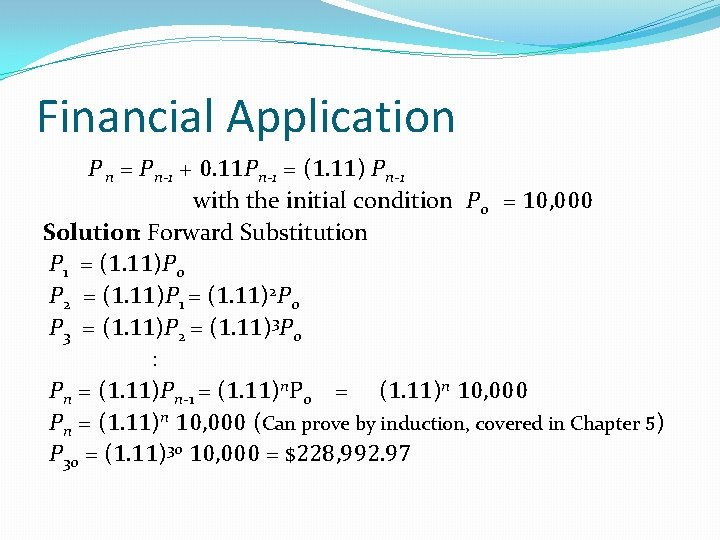Financial Application P n = P n-1 + 0. 11 Pn-1 = (1. 11) Pn-1 with the initial condition P 0 = 10, 000 Solution: Forward Substitution P 1 = (1. 11)P 0 P 2 = (1. 11)P 1 = (1. 11)2 P 0 P 3 = (1. 11)P 2 = (1. 11)3 P 0 : Pn = (1. 11)Pn-1 = (1. 11)n. P 0 = (1. 11)n 10, 000 Pn = (1. 11)n 10, 000 (Can prove by induction, covered in Chapter 5) P 30 = (1. 11)30 10, 000 = \$228, 992. 97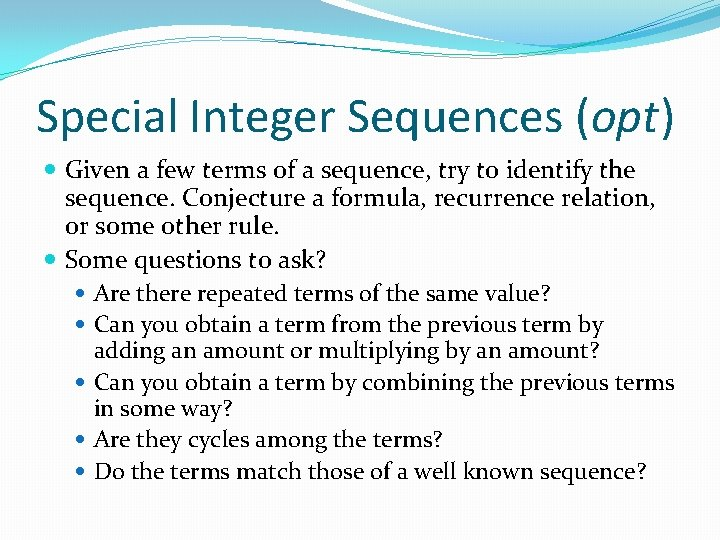Special Integer Sequences (opt) Given a few terms of a sequence, try to identify the sequence. Conjecture a formula, recurrence relation, or some other rule. Some questions to ask? Are there repeated terms of the same value? Can you obtain a term from the previous term by adding an amount or multiplying by an amount? Can you obtain a term by combining the previous terms in some way? Are they cycles among the terms? Do the terms match those of a well known sequence?Questions on Special Integer Sequences (opt) Example 1: Find formulae for the sequences with the following first five terms: 1, ½, ¼, 1/8, 1/16 Solution: Note that the denominators are powers of 2. The sequence with an = 1/2 n is a possible match. This is a geometric progression with a = 1 and r = ½. Example 2: Consider 1, 3, 5, 7, 9 Solution: Note that each term is obtained by adding 2 to the previous term. A possible formula is an = 2 n + 1. This is an arithmetic progression with a =1 and d = 2. Example 3: 1, -1, 1 Solution: The terms alternate between 1 and -1. A possible sequence is an = (− 1)n. This is a geometric progression with a = 1 and r = − 1.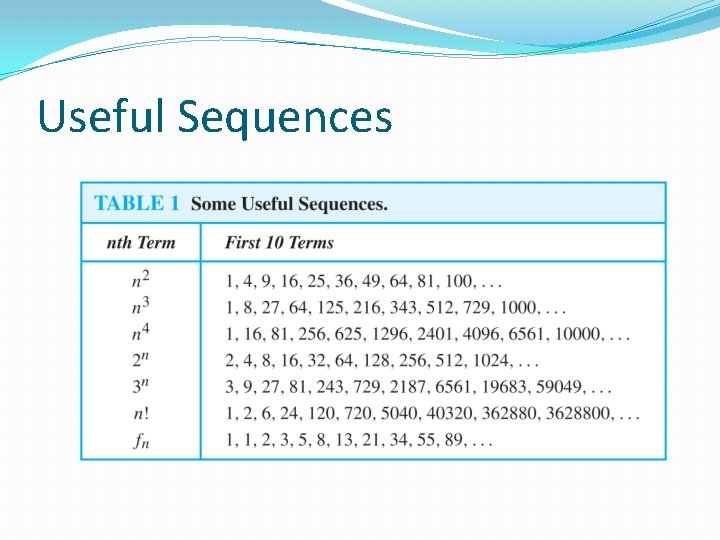Useful SequencesGuessing Sequences (optional) Example: Conjecture a simple formula for an if the first 10 terms of the sequence {an} are 1, 7, 25, 79, 241, 727, 2185, 6559, 19681, 59047. Solution: Note the ratio of each term to the previous approximates 3. So now compare with the sequence 3 n. We notice that the nth term is 2 less than the corresponding power of 3. So a good conjecture is that an = 3 n − 2.Integer Sequences (optional) Integer sequences appear in a wide range of contexts. Later we will see the sequence of prime numbers (Chapter 4), the number of ways to order n discrete objects (Chapter 6), the number of moves needed to solve the Tower of Hanoi puzzle with n disks (Chapter 8), and the number of rabbits on an island after n months (Chapter 8). Integer sequences are useful in many fields such as biology, engineering, chemistry and physics. On-Line Encyclopedia of Integer Sequences (OESIS) contains over 200, 000 sequences. Began by Neil Stone in the 1960 s (printed form). Now found at http: //oeis. org/Spuzzle. htmlInteger Sequences (optional) Here are three interesting sequences to try from the OESIS site. To solve each puzzle, find a rule that determines the terms of the sequence. Guess the rules forming for the following sequences: 2, 3, 3, 5, 10, 13, 39, 43, 172, 177, . . . Hint: Think of adding and multiplying by numbers to generate this sequence. 0, 0, 4, 9, 5, 1, 1, 0, 55, . . . Hint: Think of the English names for the numbers representing the position in the sequence and the Roman Numerals for the same number. 2, 4, 6, 30, 32, 34, 36, 40, 42, 44, 46, . . . Hint: Think of the English names for numbers, and whether or not they have the letter ‘e. ’ The answers and many more can be found at http: //oeis. org/Spuzzle. htmlSummations Sum of the terms from the sequence The notation: represents The variable j is called the index of summation. It runs through all the integers starting with its lower limit m and ending with its upper limit n.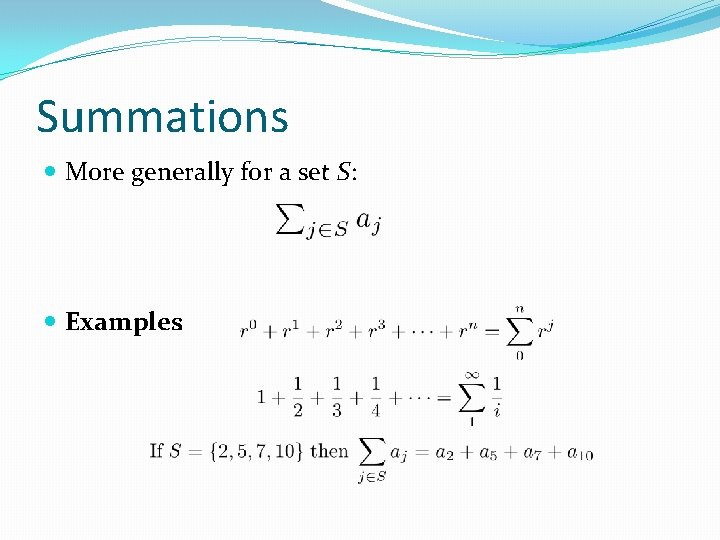Summations More generally for a set S: Examples:Product Notation (optional) Product of the terms from the sequence The notation: represents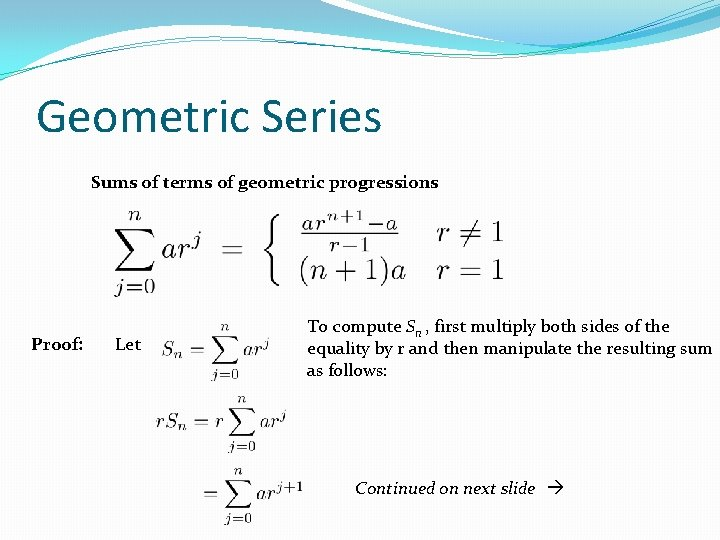Geometric Series Sums of terms of geometric progressions Proof: Let To compute Sn , first multiply both sides of the equality by r and then manipulate the resulting sum as follows: Continued on next slide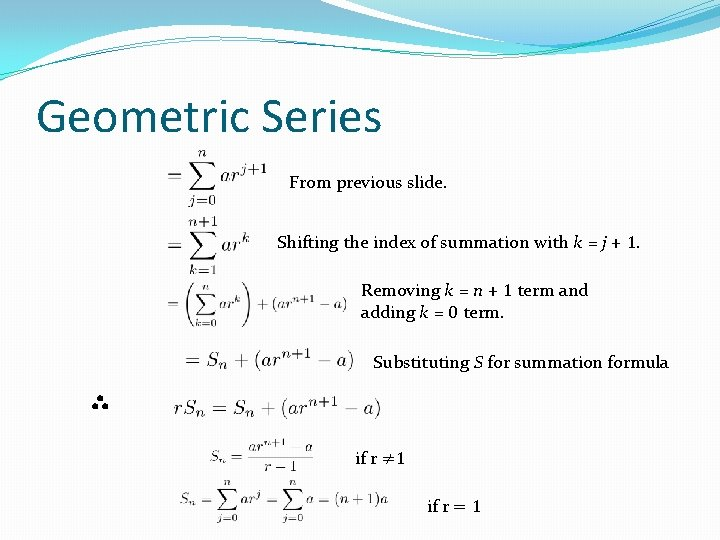Geometric Series From previous slide. Shifting the index of summation with k = j + 1. Removing k = n + 1 term and adding k = 0 term. Substituting S for summation formula ∴ if r ≠ 1 if r = 1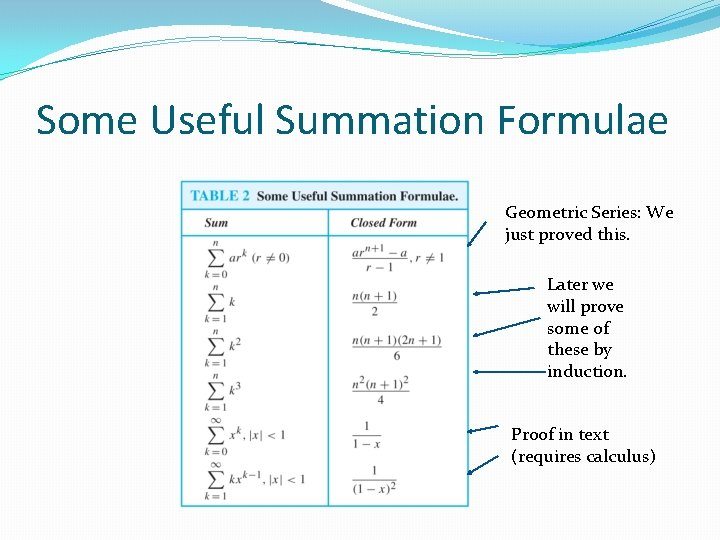Some Useful Summation Formulae Geometric Series: We just proved this. Later we will prove some of these by induction. Proof in text (requires calculus)